#Wolfram Summer School

# Alumni# Luan Ozelim

Summer School

Class of 2013

## Bio

In 2012, Luan received a BSc degree in Civil Engineering from the University of Brasilia, Brazil, and is currently pursuing a PhD degree in Geotechnics at the same university. Throughout his undergraduate studies, even though deeply interested in Engineering, Mathematics and Applied Physics stood out as favorite areas of study. He has worked on the theory and application of special functions to science, exploring how this amazing class of functions can be applied to analytically solve problems in Mathematical Statistics, Physics, and Engineering. A great part of his work involves generalized hypergeometric functions, such as the H-function. Concerning the Applied Physics side of his research interests, modeling flow through porous media plays a central role. By the end of his undergraduate course, he was introduced to cellular automata philosophy, being instantaneously amazed by the latter. In fact, his undergraduate project was related to the usage of elementary cellular automata to model contaminant transport through soils, which ultimately led to the definition of the iota-delta function. His PhD dissertation is related to the role the iota-delta function plays in flow modelling by means of lattice gas automata and lattice Boltzmann techniques. In his free time, staying with his family, listening to good music, and watching movies are his preferred activities.

## Project: Classifying Elementary Cellular Automata by Means of Their Iota-Delta Function Representation

One of the most interesting characteristics of dynamical systems is their complexity. Thus, mathematicians, computer scientists and physicists have always tried to establish ways of ranking dynamical systems in terms of a given measure of complexity (Wolfram, 2002).

The present project is intended to investigate a classification system for ECA based on the iota-delta function representation of their evolution rules. The iota-delta function can be defined as (Ozelim et al., 2013a and 2013b):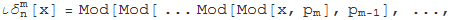in which Mod[o,p] denotes the modulus operator, which gives the rest of the division of o by p if o is greater than p or o itself; otherwise, m and n are parameters of the iota-delta function, pm is the mth prime number and pi[n] stands for the prime counting function which gives the number of primes less than or equal to n. The value of n determines how many states the automata generated have. Thus, for binary automaton, n = 2, for ternary ones, n = 3, for quaternary ones, n = 4 and so on. The iota-delta function is periodic with period length of pi[n].

In short, every ECA rule can be represented by means of the following evolution equation (Ozelim et al., 2013a):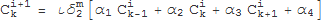in which Cki+1 stands for the value of a given cell in terms of itself (Cki) and its immediate left and right neighbors in the previous step (Ck-1i and Ck+1i, respectively). For example, the evolution of rule 30 can be given as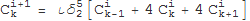while the evolution of rule 110 can be represented in terms of the iota-delta function as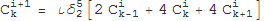It is worth noticing that due to the structure of the iota-delta function, the coefficients alphaj, j = 1, …, 4; range from 0 to pm -1. This constraint leads to a total of pm4 possible evolution rules of the type of Eq. (1) for a fixed value of m.

The classification is to be accomplished by means of large-scale computational experiments. At first, a Mathematica routine will be written in order to evaluate each of the pm4 possible evolution rules. Subsequently, for a given m, each tuple {alpha1,alpha2,alpha3,alpha4} will be matched to a given ECA rule by means of a rule numbering function. Following that, rules will be clustered in terms of frequency of appearance. Finally, the clusters formed will be treated as classes and their properties will be studied.

If time allows, some simulations regarding the iota-delta classification of binary CA with 4 neighbors are to be carried out. Also, the role of the iota-delta function as an equivalent to every Boolean function is to be verified.

## Favorite Four-Color, Four State Turing Machine

Rule 3764655765361914539851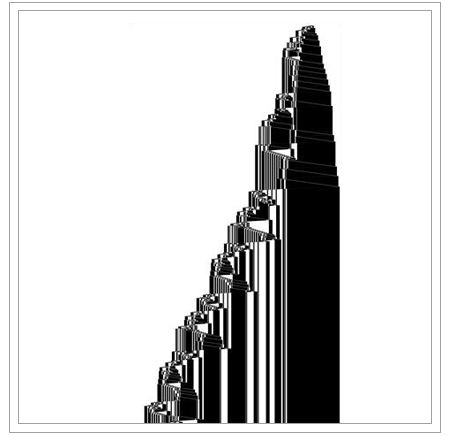• 21st Annual Wolfram Summer School
• Bentley University, Waltham, MA, USA
• June 25–July 15, 2023